RRB JE CE (CBT I) Mock Test- 2

# RRB JE CE (CBT I) Mock Test- 2 - Civil Engineering (CE)

Test Description

## 100 Questions MCQ Test - RRB JE CE (CBT I) Mock Test- 2

RRB JE CE (CBT I) Mock Test- 2 for Civil Engineering (CE) 2023 is part of Civil Engineering (CE) preparation. The RRB JE CE (CBT I) Mock Test- 2 questions and answers have been prepared according to the Civil Engineering (CE) exam syllabus.The RRB JE CE (CBT I) Mock Test- 2 MCQs are made for Civil Engineering (CE) 2023 Exam. Find important definitions, questions, notes, meanings, examples, exercises, MCQs and online tests for RRB JE CE (CBT I) Mock Test- 2 below.
Solutions of RRB JE CE (CBT I) Mock Test- 2 questions in English are available as part of our course for Civil Engineering (CE) & RRB JE CE (CBT I) Mock Test- 2 solutions in Hindi for Civil Engineering (CE) course. Download more important topics, notes, lectures and mock test series for Civil Engineering (CE) Exam by signing up for free. Attempt RRB JE CE (CBT I) Mock Test- 2 | 100 questions in 90 minutes | Mock test for Civil Engineering (CE) preparation | Free important questions MCQ to study for Civil Engineering (CE) Exam | Download free PDF with solutions
 1 Crore+ students have signed up on EduRev. Have you?
RRB JE CE (CBT I) Mock Test- 2 - Question 1

### In a school, 1000 chocolates were distributed in such a way that each student gets chocolates equal to 10% of the total students. Find the number of chocolates that each student gets

Detailed Solution for RRB JE CE (CBT I) Mock Test- 2 - Question 1
Let the number of students = s

10 x s x s/100 = 1000

s2 = 10,000 s = 100

Each student got 10 x 100/100

=> 10 chocolates

RRB JE CE (CBT I) Mock Test- 2 - Question 2

### If the cost price of 12 pen is same as the selling price of 15 pen. What is the percentage of gain or loss?

Detailed Solution for RRB JE CE (CBT I) Mock Test- 2 - Question 2
CP of 12 pen = SP of 15 pen CP x 12 = SP x 15

=> CP/SP - 5 / 4…(1)

From equation (1) we can see that CP >SP, therefore there will be loss. Let the CP = 5 and SP = 4 Then Loss = 1

Loss% = loss/CP x 100

= 1/5 x 100

= 20% loss

RRB JE CE (CBT I) Mock Test- 2 - Question 3

### The following table represents marks obtained by four students in five subjects and maximum marks of each subject.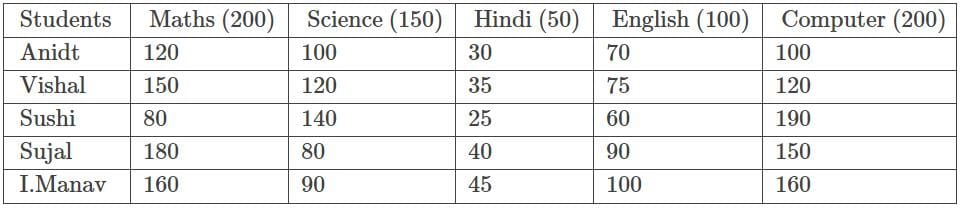Q. Find the respective ratio of overall percentage of marks obtained by Ankil in all the five subjects and overall percentage of marks obtained by Sulal in all the five subjects.

Detailed Solution for RRB JE CE (CBT I) Mock Test- 2 - Question 3
Total marks in all subjects = 200 + 150 + 50 + 100 + 200 = 700

Overall percentage of marks obtained by Ankit in all the five subjects

= (120 + 100 + 30 + 70 + 100)/700 x 100

= 420/700 x 100

= 60%

Overall percentage of marks obtained by Sujal in all the five subjects

= (180 + 80 + 40 + 90 + 150)/700 x 100

= 540/700 x 100

= 540/7%

Required ratio = 60 : 540/7

= 420 : 540

= 7 : 9

RRB JE CE (CBT I) Mock Test- 2 - Question 4

Area of rhombus is (7 + 6.5√5) cm2 and length of its one diagonal is (3 + 2√5) cm, then what is the length of its second diagonal?

Detailed Solution for RRB JE CE (CBT I) Mock Test- 2 - Question 4
Area of rhombus = (First diagonal x Second diagonal) / 2

2 x Area of rhombus = (First diagonal x Second diagonal)

Let its second diagonal = (a + b√5) cm

(14 + 13√5) = (3 + 2√5) x (a + b√5)

(14 + 13√5) = (3a + 3b√5 + 2a√5 + 10b)

After comparing-

(3a + 10b) = 14 ...... (1)

(2a + 3b) = 13 ....... (2)

From (1) and (2)-

a = 8 and b = -1

Length of second diagonal = (a + b√5) = (8 - √5) cm

RRB JE CE (CBT I) Mock Test- 2 - Question 5

Solve the following expression: (∛42875 ÷ √49) + (152 - 21 x 10) - √625.

Detailed Solution for RRB JE CE (CBT I) Mock Test- 2 - Question 5
= (∛42875 ÷ √49) + (152 - 21 x 10) - √625

= (35 ÷ 7) + (225 - 210) - 25

= 5 + 15 - 25

= -5

RRB JE CE (CBT I) Mock Test- 2 - Question 6

A set of 7 pipes can fill 70% of a vessel in 7 minutes. Another set of 5 pipes can fill 2/5th of the vessel in 5 minutes. A third set of 10 pipes can empty 1/3rd of the tank in 10 minutes. How much time (in minutes) will it take to fill the entire tank if all the pipes are opened at the same time?

Detailed Solution for RRB JE CE (CBT I) Mock Test- 2 - Question 6
7 pipes of first set can fill 7/10th of the vessel in 7 minutes.

Hence, 7 pipes of first set can fill 1/10th of the vessel in 1 min.

5 pipes of second set can fill 2/5th of the vessel in 5 minutes

Hence, 5 pipes of second set can fill 2/25th of the vessel in 1 min.

10 pipes of third set can empty 1/3rd of the vessel in 10 minutes.

Hence, 10 pipes of third set can empty 1/30th of the vessel in 1 min

So, if all the pipes are open in one minute 1/10 + 2/25 - 1/30 = 11/75th of the vessel is filled up.

Hence, to fill up the whole vessel, time required= 75/11 minutes.

RRB JE CE (CBT I) Mock Test- 2 - Question 7

What is the mass number of Gold?

Detailed Solution for RRB JE CE (CBT I) Mock Test- 2 - Question 7
The mass number of Gold is 197. Mass number is the sum of the numbers of protons and neutrons present in the nucleus of an atom.

Mass numbers of some common elements-

Neon- 20

Sodium- 23

Silver- 108

RRB JE CE (CBT I) Mock Test- 2 - Question 8

If an article is sold for a gain of 7% instead of selling it at a loss of 13%, a trader gets Rs.1080 more. What is the selling price of the article, when the article is sold at a profit of 25%?

Detailed Solution for RRB JE CE (CBT I) Mock Test- 2 - Question 8
Let cost price of article be Rsx (13 + 7)% of x = 1080

20% of x = 1080

x = 5400

SP = 125% of 5400 = 6750

RRB JE CE (CBT I) Mock Test- 2 - Question 9

There are total 10 students whose marks in an exam are 45, 65, 56, (P + 16), (2P + 3), 78, 24, (4P - 8), 44 and 48 respectively and mean marks of first 5 students is 49, then what is mean marks of last 5 students?

Detailed Solution for RRB JE CE (CBT I) Mock Test- 2 - Question 9
Mean marks of first 5 students = [45 + 65 + 56 + (P + 16) + (2P + 3)]/5 = 49

185 + 3P = 245

3P = 60

P = 20

Mean marks of first 5 students = [78 + 24 + (4P - 8) + 44 + 48]/5 = (186 + 4P)/5 = 266/5

= 53.2

RRB JE CE (CBT I) Mock Test- 2 - Question 10

Select the odd one out: (6 x 10-3), √[(52 + 11) x 10-6], (0.06 ÷ 102) and √0.000036.

Detailed Solution for RRB JE CE (CBT I) Mock Test- 2 - Question 10
(6 x 10-3) = 0.006

√[(52 + 11) x 10-6] = 6 x 10-3 = 0.006

(0.06 ÷ 102) = 0.0006

√0.000036 = 0.006

RRB JE CE (CBT I) Mock Test- 2 - Question 11

There is a fraction such that if we add 11/30 to it then it gets reverted. Further if we subtract 1 from numerator then the fraction becomes 2/3. What is the fraction?

Detailed Solution for RRB JE CE (CBT I) Mock Test- 2 - Question 11
Let the fraction = m/n

According to the 1st condition; m/n + 11/30= n/m ...(1)

According to the 2nd condition; (m - 1)/n = ⅔

=> 3m - 3 = 2n

=> 3m - 2n = 3 ...(2)

By solving equation 1,

=> 30m2 + 11mn - 30n2 = 0

=> (6m - 5n)(5m + 6n) = 0

we get the value of m/n = 5/6, -6/5

applying condition(2), m/n = (5 - 1)/6 = 4/6 = 2/3

Hence the required fraction = m/n = 5/6

RRB JE CE (CBT I) Mock Test- 2 - Question 12

How many among the following numbers are Irrational number: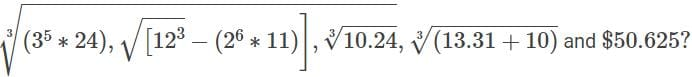Detailed Solution for RRB JE CE (CBT I) Mock Test- 2 - Question 12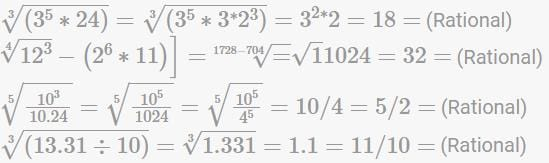RRB JE CE (CBT I) Mock Test- 2 - Question 13

Ramesh and Suresh invested same amount of money in two banks for 2 years which offer interest at the same rate, but Ramesh invested in a bank which offers compound interest and Suresh invested in a bank which offers simple interest. After two years Ramesh got Rs. 525 as interest and Suresh got Rs. 500 as interest. What is the rate of interest offered by the banks?

Detailed Solution for RRB JE CE (CBT I) Mock Test- 2 - Question 13
In case of simple interest we get the same amount of interest each year.

Hence amount of interest Suresh will get in first year = 500/2 = Rs. 250

If the same amount is invested and interest rate is same then in first year the interest obtained is same whether it is simple interest or compound interest.

Hence compound interest obtained in 1st year = Rs. 250

Therefore amount of interest obtained in the 2nd year = Rs. (525 - 250) = Rs. 275

Hence rate of interest = 100 x (275 - 250)/250 = 10%

RRB JE CE (CBT I) Mock Test- 2 - Question 14

Line graph given below shows the total number of presence of employees in two different companies P and Q on five different working days from Monday to Friday.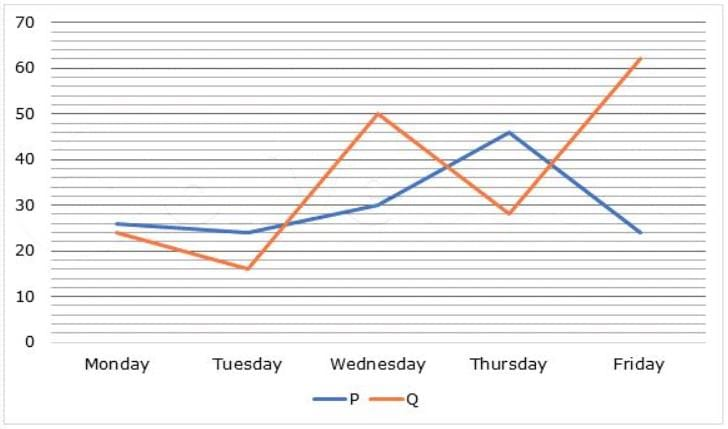Q. What is the sum of the average number of presence of employees in company P and average number of presence of employees in company Q on all the five working days?

Detailed Solution for RRB JE CE (CBT I) Mock Test- 2 - Question 14
Average number of presence of employees in company P on all the five working days = 26 + 24 + 30 + 46 + 24 = 150

Average = 150/5 = 30

Average number of presence of employees in company Q on all the five working days = 24 + 16 + 50 + 28 + 62 = 180

Average = 180/5 = 36

Required sum = 30 + 36 = 66

RRB JE CE (CBT I) Mock Test- 2 - Question 15

If [(0.09)2 x (0.3)8 x (0.027) x 1012] / [312 x (0.3)x] = 1, then what is the value of x?

Detailed Solution for RRB JE CE (CBT I) Mock Test- 2 - Question 15
[(0.09)2 x (0.3)8 x (0.027) x 1012] / [312 x (0.3)x] = 1

[(0.09)2 x (0.3)8 x (0.027) x 1012] = [312 x (0.3)x]

[(0.3)4 x (0.3)8 x (0.3)3] = [(0.3)12 x (0.3)x]

(0.3)15 / (0.3)12 = (0.3)x

(0.3)3 = (0.3)x

x = 3

RRB JE CE (CBT I) Mock Test- 2 - Question 16

In one revolution, the object travels______ distance.

Detailed Solution for RRB JE CE (CBT I) Mock Test- 2 - Question 16
In one revolution, the object travels 2πr distance. Revolution means a circular motion around an axis located outside the object. E.g., Rounding a curve in a car, merry go round, etc.
RRB JE CE (CBT I) Mock Test- 2 - Question 17

Sita buys a parker pen at Rs. 100 and sell it to Geeta at Rs. 120. Geeta sells this pen to Radha at a price of Rs. 80. Radha sells it back to Sita at Rs. 90. Sita sells it to Roma at Rs. 150. What is Sita's profit/loss %?

Detailed Solution for RRB JE CE (CBT I) Mock Test- 2 - Question 17
C.P. of pen for Sita = Rs 100

S.P. of pen for Sita = Rs 150 - 90 + 120 = 180

Profit % = [(180 - 100)/100] x 100 = 80%

RRB JE CE (CBT I) Mock Test- 2 - Question 18

Mahesh is travelling at a distance of 1200 km in Japan by Bullet train. Due to some technical fault in the engine of the train, speed of the train was reduced by 100 km/hr thus overall time of his journey increased by 24 minutes. What was the normal speed of the bullet train?

Detailed Solution for RRB JE CE (CBT I) Mock Test- 2 - Question 18
Let the normal speed of the bullet train is N km/hr and the corresponding time taken is A hours.

Hence; 1200/N = A .. (1)

According to the 2nd condition; 1200/(N - 100) = A + 2/5 .. (2)

Equation (2) - Equation (1); 1200/(N - 100) - 1200/N = 2/5

If we will try to solve this equation then it will become too lengthy. Hence such questions we should solve by using the options.

Out of the given options, option (c) satisfies above equation.

Hence; N = 600 km/hr

RRB JE CE (CBT I) Mock Test- 2 - Question 19

If 4x - 1/3x = 9, then what is the value of (144x4 - 1)/(27x2 + 2x)?

Detailed Solution for RRB JE CE (CBT I) Mock Test- 2 - Question 19
(12x2 - 1)/3x = 9

=> (12x2 - 1)/3x = 9

=> 12x2 - 1 = 27x ------- (i)

Now,

(144x4 - 1)/(27x2 + 2x)

= [(12x2 - 1)(12x2 + 1)]/[x(27x + 2)] ------- [(a2 - b2 = (a - b)(a + b)]

= [27x(27x -1 + 1 +1)]/[x(27x + 2)] ---- {Putting the value of equation (i)]

= [27x(27x + 2)]/[x(27x + 2)]

= 27

RRB JE CE (CBT I) Mock Test- 2 - Question 20

What is the simplified value of (cos3 x/sin x) + (cos2 x.cot3 x)?

Detailed Solution for RRB JE CE (CBT I) Mock Test- 2 - Question 20
cos3 x/sin x + cos2 x cot3 x

= (cos3 x/sin3 x) sin2 x + cos2 x cot3 x

= cot3 x sin2 x + cos2 x cot3 x

= cot3 x (sin2 x + cos2 x)

= cot3 x

RRB JE CE (CBT I) Mock Test- 2 - Question 21

If 25% of (A + B) is 30% of (A - B), then B is what percent of A?

Detailed Solution for RRB JE CE (CBT I) Mock Test- 2 - Question 21
=> 25/100(A + B) = 30/100(A - B)

=> 5A + 5B = 6A - 6B => A = 11B

=> Let p% of B is equal to A

=> p/100 x B = A

=> But B = 11A

RRB JE CE (CBT I) Mock Test- 2 - Question 22

What is the ∠SRO if O is the centre of circle, ∠SPO = 550 and ∠POR = 1150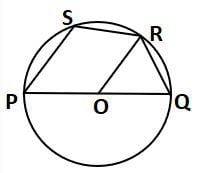Detailed Solution for RRB JE CE (CBT I) Mock Test- 2 - Question 22
In quadrilateral PQRS, ∠SRQ = 180 − ∠SPO = 180 − 55 = 125

[Sum of opposite angle of a cyclic quadrilateral is 1800.]

∠ROQ = 180 − ∠POR = 1800 − 115 = 65

In △QOR, ∠OQR = ∠ORQ [Angles opposite or same side] ∠ROQ + ∠OQR + ∠ORQ = 180

650 + ∠ORQ + ∠ORQ = 180

∠ORQ = (1800 − 65)/2 = 57.5

RRB JE CE (CBT I) Mock Test- 2 - Question 23

A number 135 is split into three parts in such a way that all the three parts are in increasing Arithmetic Progression. The Product of the two largest parts is 2160, then what is the ratio of the smallest part to the highest part?

Detailed Solution for RRB JE CE (CBT I) Mock Test- 2 - Question 23
Let the three numbers are: (a - d), a, (a + d) respectively.

According to questions:

a(a + d) = 2160 ...... (1)

(a - d) + a + (a + d) = 135

a = 45

From equation (1)

45(45 + d) = 2160

(45 + d) = 48

d = 3

Required ratio = (a - d) : (a + d) = 42 : 48 = 7 : 8

RRB JE CE (CBT I) Mock Test- 2 - Question 24

Simplify the value of 3[{1/(√9 - √8)} - {1/(√9 + √8)}] + (2 - 3√2)2.

Detailed Solution for RRB JE CE (CBT I) Mock Test- 2 - Question 24
= 3[{1/(√9 - √8)} - {1/(√9 + √8)}] + (2 - 3√2)2

= 3[{(√9 + √8) - (√9 - √8)}/(√9 - √8)(√9 + √8)] + (2 - 3√2)2

= 3[2√8] + (2 - 3√2)2

= 6√8 + (4 + 18 - 12√2)

= 12√2 + (22 - 12√2)

= 22

RRB JE CE (CBT I) Mock Test- 2 - Question 25

There are 7 students in a class whose weights are: 54 kg, 45 kg, 48 kg, A kg, 42 kg, 50 kg and 55 kg. If the mean of their weights is 49 kg, then what is the median of their weights?

Detailed Solution for RRB JE CE (CBT I) Mock Test- 2 - Question 25
Mean of their weights = (54 + 45 + 48 + A + 42 + 50 + 55)/7 = 49

294 + A = 343

A = 49 kg

Arrange the weights in ascending order = 42, 45, 48, 49, 50, 54, 55

Median of their weights = 4th term in series = 49 kg

RRB JE CE (CBT I) Mock Test- 2 - Question 26

The LCM of two numbers is 2376, and their HCF is 22. Find their difference, if the sum of numbers is 682.

Detailed Solution for RRB JE CE (CBT I) Mock Test- 2 - Question 26
Let the number be 22x and 22y

22x + 22y = 682

x + y = 31 → (1)

22x x 22y = 2376 x 22

xy = 108 → (2)

Solving (1) and (2), we get

x = 27 and y = 4

Difference = 22x - 22y

= 22(27 - 4)

= 22 x 23

= 506

RRB JE CE (CBT I) Mock Test- 2 - Question 27

60 liters of first mixture of milk and water is mixed with another 40 liters mixture of milk and water. After mixing the mixture, the mixture is sold at the cost of pure milk, then what is the percent profit gained if the ratio of milk to water in the first and second mixture is 7: 5 and 3: 5, respectively? Given below are the steps involved. Arrange them in sequential order.

(A) Amount of milk and water in first mixture is 60 x (7/12) = 35 liters and 60 x (5/12) = 25 liters respectively.

(B) Total amount of milk and water is final mixture is (35 + 15) = 50 liters and (25 + 25) = 50 liters respectively.

(C) Percent profit earned = [(100 - 50)/50] x 100 = 100%

(D) Amount of milk and water in second mixture is 40 x (3/8) = 15 liters and 40 x (5/8) = 25 litres respectively.

(E) Cost price of final mixture = 50 x 1 = 50 and selling price of final mixture = 100 x 1 = 100

Detailed Solution for RRB JE CE (CBT I) Mock Test- 2 - Question 27
The correct arrangement is:

(A) Amount of milk and water in first mixture is 60 x (7/12) = 35 litres and 60 x (5/12) = 25 liters respectively.

(D) Amount of milk and water in second mixture is 40 x (3/8) = 15 liters and 40 x (5/8) = 25 liters respectively.

(B) Total amount of milk and water is final mixture is (35 + 15) = 50 litres and (25 + 25) = 50 liters respectively.

(E) Cost price of final mixture = 50 x 1 = 50 and selling price of final mixture = 100 x 1 = 100

(C) Per cent profit earned = [(100 - 50)/50] x 100 = 100%

RRB JE CE (CBT I) Mock Test- 2 - Question 28

If cosec θ = x + 1/4x, then the value of cosec θ + cot θ is:

Detailed Solution for RRB JE CE (CBT I) Mock Test- 2 - Question 28
Let cosec θ + cot θ = k

⇒ cosec θ - cot θ = 1/k

2 cosec θ = k + 1/k = 2(x + 1/4x) = k + 1/k

⇒ x + 1/2x = k + 1/k ⇒ k = 2x

RRB JE CE (CBT I) Mock Test- 2 - Question 29

At present, the sum of ages of R and K is 63 years. The ratio of their ages after 7 years will be 7: 4 , what is the present age of R?

Detailed Solution for RRB JE CE (CBT I) Mock Test- 2 - Question 29
Let the present age of R is x

⇒ present age of K is 63 - x

7 years later

R's age = x + 7

K's age = 63 - x + 7= 70 - x

⇒ (x + 7) / (70 - x) = 7 / 4

⇒ 4x + 28= 490 - 7x

⇒ 11x = 462

⇒ x = 42 years

therefore present age of R = 42 years

RRB JE CE (CBT I) Mock Test- 2 - Question 30

If 5 girls can embroider a dress in 9 days, then the number of days taken by 3 girls will be _____.

Detailed Solution for RRB JE CE (CBT I) Mock Test- 2 - Question 30
Days taken by 5 girls to embroider a dress = 9 days

Days taken by 1 girl to embroider a dress = 9 x 5 days

Days taken by 3 girls to embroider a dress = 9 × 5/3 days = 15 days

RRB JE CE (CBT I) Mock Test- 2 - Question 31

In a certain code language, ‘POETRY’ is written as ‘QONDSQX’ and ‘OVER’ is written as ‘PNUDQ.’ How is ‘MORE’ written in that code language?

Detailed Solution for RRB JE CE (CBT I) Mock Test- 2 - Question 31
AS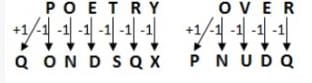SIMILARLY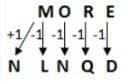RRB JE CE (CBT I) Mock Test- 2 - Question 32

Which of the answer figure is embedded in the problem figure?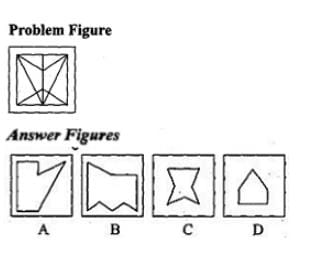Detailed Solution for RRB JE CE (CBT I) Mock Test- 2 - Question 32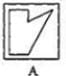answer figure A is embedded in problem figure.

RRB JE CE (CBT I) Mock Test- 2 - Question 33

Arrange the words given below in a meaningful sequence.

1. Key

2. Door

3. Lock

4. Room

5. Switch on

Detailed Solution for RRB JE CE (CBT I) Mock Test- 2 - Question 33
The correct order is:

1. Key

3. Lock

2. Door

4. Room

5. Switch on

RRB JE CE (CBT I) Mock Test- 2 - Question 34

Which of the given answer figure will be the correct mirror image of the problem figure?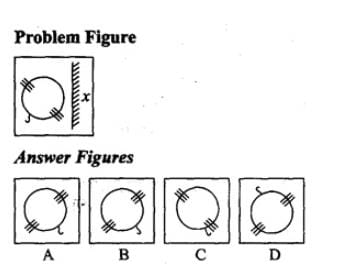Detailed Solution for RRB JE CE (CBT I) Mock Test- 2 - Question 34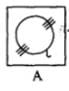A is the correct mirror image.

RRB JE CE (CBT I) Mock Test- 2 - Question 35

DIRECTIONS: Which of the words in the following options can be formed using the letter of the given word?

DETERMINATION

Detailed Solution for RRB JE CE (CBT I) Mock Test- 2 - Question 35
There is no ‘C’ in the given word.So, DECLARATION cannot be formed.

There is no ‘L’ in the given word.So, NATIONAL cannot be formed.

There is no ‘V’ in the given word.So, DEVIATION cannot be formed.

RRB JE CE (CBT I) Mock Test- 2 - Question 36

DIRECTIONS: Solve the following letter series.

adb _ ac _ da _ cddcb _ dbc _ cbda

Detailed Solution for RRB JE CE (CBT I) Mock Test- 2 - Question 36
The series is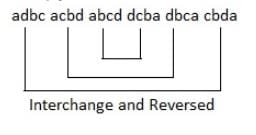RRB JE CE (CBT I) Mock Test- 2 - Question 37

DIRECTIONS: Choose the odd one out from the given alternatives.

Detailed Solution for RRB JE CE (CBT I) Mock Test- 2 - Question 37
Second of all, the word first is the opposite, but in the second option, this format was not followed.
RRB JE CE (CBT I) Mock Test- 2 - Question 38

DIRECTIONS: Choose the odd one out from the given alternatives.

Detailed Solution for RRB JE CE (CBT I) Mock Test- 2 - Question 38
Except PRTSQ in all other groups, the consecutive letters are written alternately.
RRB JE CE (CBT I) Mock Test- 2 - Question 39

Arrange the following words according to the English Dictionary.

(1) Save

(2) Sausage

(3) Saviour

(4) Savour

(5) Savage

Detailed Solution for RRB JE CE (CBT I) Mock Test- 2 - Question 39
Sausage, Savage, Save, Saviour, Savour
RRB JE CE (CBT I) Mock Test- 2 - Question 40

DIRECTIONS: Find the odd one out from the given alternatives.

Detailed Solution for RRB JE CE (CBT I) Mock Test- 2 - Question 40
Except 75 all other numbers are perfect cubes.

343 = 7 × 7 × 7

64 = 4 × 4 × 4

27 = 3 × 3 ×3

RRB JE CE (CBT I) Mock Test- 2 - Question 41

Select the correct combination of mathematical signs to replace * signs and to balance the given equation:

15 * 24 * 3 * 6 * 17

Detailed Solution for RRB JE CE (CBT I) Mock Test- 2 - Question 41
Given that

15 * 24 * 3 * 6 * 17

After applying, + ÷ – =

15 + 24 ÷ 3 – 6 = 17

⇒ 15 + 8 – 6 = 17

⇒ 23 – 6 = 17

⇒ 17 = 17.

RRB JE CE (CBT I) Mock Test- 2 - Question 42

DIRECTIONS: In the question given below, an unfolded dice is given in the left side while in the right side, four answer choices are given in the form of complete dices. You have to select the correct answer choice(s) which is are formed by folding the unfolded dice.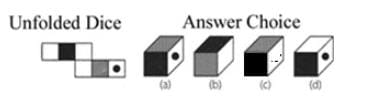Detailed Solution for RRB JE CE (CBT I) Mock Test- 2 - Question 42
According to the unfolded dice,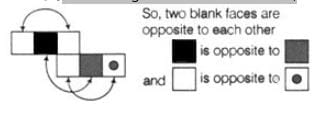Hence, only option (d) is correct.

RRB JE CE (CBT I) Mock Test- 2 - Question 43

Pointing to a girl in a photograph, Sunita said, "She is the mother of Renu, whose father is my son". How is Sunita related to that girl?

Detailed Solution for RRB JE CE (CBT I) Mock Test- 2 - Question 43
If the woman in the picture is Renu's mother, and Renu's father is Sunita's son, that means Sunita is the mother-in-law of the woman in the photo.
RRB JE CE (CBT I) Mock Test- 2 - Question 44

Rihana walks 3 km from her house in the East direction to reach the bus stop. She takes a bus and goes 17 km ahead. After getting down from the bus, she walks 2 km on the left side, to reach the spot where she meets Santa. Santa and Rihana take a right from that point and travel 3 km to reach the market place. After shopping, they turn right from the market place and walk 2km to reach Sanita house for a cup of tea. What is the straight line distance between Rihana’s house and Sanita house?

Detailed Solution for RRB JE CE (CBT I) Mock Test- 2 - Question 44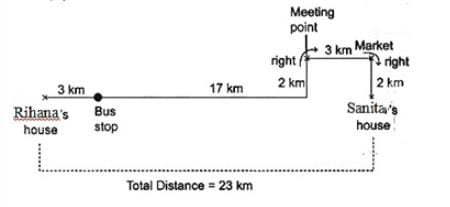RRB JE CE (CBT I) Mock Test- 2 - Question 45

Select the correct order of the letters as indicated by the numbers to form a meaningful word.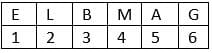Detailed Solution for RRB JE CE (CBT I) Mock Test- 2 - Question 45
GAMBLE

Hence option D is correct.

RRB JE CE (CBT I) Mock Test- 2 - Question 46

DIRECTIONS: Select the related word from the given alternatives.

BDGK : CEHL :: ???? : DFIM

Detailed Solution for RRB JE CE (CBT I) Mock Test- 2 - Question 46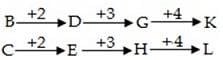SAME WAY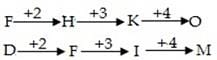RRB JE CE (CBT I) Mock Test- 2 - Question 47

DIRECTIONS: Which of the words in the following options can be formed using the letter of the given word?

CORRESPONDING

Detailed Solution for RRB JE CE (CBT I) Mock Test- 2 - Question 47
There is no ‘T’ in the given word. So, CORRECT and REPENT cannot be formed.

There is only one ‘S’ in the given word. So, RESPONSE cannot be formed.

RRB JE CE (CBT I) Mock Test- 2 - Question 48

DIRECTIONS: What will come in place of question marks?

20, 20, 19, 16, 17, 13, 14, 11, ?, ?

Detailed Solution for RRB JE CE (CBT I) Mock Test- 2 - Question 48
There are two series.

1st series: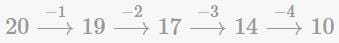2nd series: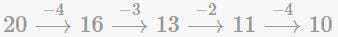RRB JE CE (CBT I) Mock Test- 2 - Question 49

DIRECTIONS: What should come at the place of question mark?

N5V, K7T, ?, E14P, B19N

Detailed Solution for RRB JE CE (CBT I) Mock Test- 2 - Question 49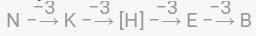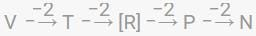RRB JE CE (CBT I) Mock Test- 2 - Question 50

DIRECTIONS: In the question below are given few statements followed by few conclusions. You have to take the given statements to be true even if they seem to be at variance from commonly known facts. Read all the conclusions and then decide which of the given conclusion logically follows from the given statements disregarding commonly known facts.

Statements:

All kings are queens.

Some queens are soldiers.

All soldiers are strong.

Conclusions:

I. Some strong are kings.

II. Some queens are strong.

Detailed Solution for RRB JE CE (CBT I) Mock Test- 2 - Question 50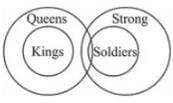∴ only Conclusion II follows

RRB JE CE (CBT I) Mock Test- 2 - Question 51

In the question, select the related digit from the given alternatives.

24 : 60:: 120:conclusion

Detailed Solution for RRB JE CE (CBT I) Mock Test- 2 - Question 51
24 x 5 = 120

Similarly,

60 x 5 = 300

RRB JE CE (CBT I) Mock Test- 2 - Question 52

How many triangles are there in the following figure?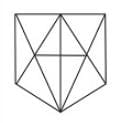Detailed Solution for RRB JE CE (CBT I) Mock Test- 2 - Question 52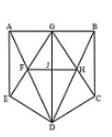Thus there are 23 triangles.

AEF, AGF, DEF,

AED, AEG, GJF,

GJH, GFH, FGD,

FDH, FJD, JHD,

GHD, GBH, BHC,

GBC, BCD, DCH,

AGD, BGD

RRB JE CE (CBT I) Mock Test- 2 - Question 53

In a certain code language 'POULTRY' is written as 'PRQXNVTY'. How is 'TREASON' written in that code?

Detailed Solution for RRB JE CE (CBT I) Mock Test- 2 - Question 53
There is one letter more in the code as compared to the letters of the given word. The pattern of coding is as follows.

As,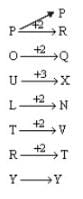SIMILARLY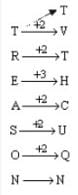RRB JE CE (CBT I) Mock Test- 2 - Question 54

Solve the given letter series:

W64X49, M100N81, O144P121, ?

Detailed Solution for RRB JE CE (CBT I) Mock Test- 2 - Question 54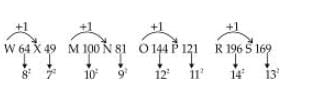RRB JE CE (CBT I) Mock Test- 2 - Question 55

DIRECTIONS: The following questions are based on the diagram given below.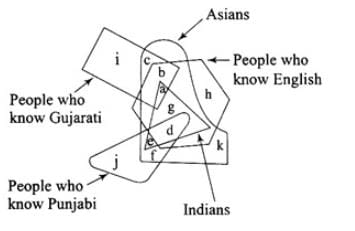The non-Asians who know English are represented by:

Detailed Solution for RRB JE CE (CBT I) Mock Test- 2 - Question 55
h, because all other comes under Asians.

Hence option D is correct.

RRB JE CE (CBT I) Mock Test- 2 - Question 56

Which of the following rivers does not make an estuary?

Detailed Solution for RRB JE CE (CBT I) Mock Test- 2 - Question 56
Mahanadi River does not make an estuary. An estuary is a partially enclosed coastal body of brackish water with one or more rivers or streams flowing into it, and with a free connection to the open sea. Estuaries form a transition zone between river environments and maritime environments.
RRB JE CE (CBT I) Mock Test- 2 - Question 57

Which of the following country's government passed the "Hindu Marriage Bill 2016"?

Detailed Solution for RRB JE CE (CBT I) Mock Test- 2 - Question 57
• Hindu Marriage Bill tabled in Pakistan’s National Assembly.

• The bill was approved by the standing committee in 2016.

• The bill is expected to put an end to the practice of abduction of married Hindu women.

• The bill is also expected to curtail forced conversions because it will allow the registration of Hindu marriages in the relevant government departments.

RRB JE CE (CBT I) Mock Test- 2 - Question 58

Who among the following has been appointed as part-time chairman of the YES BANK?

Detailed Solution for RRB JE CE (CBT I) Mock Test- 2 - Question 58
The Reserve Bank of India has approved the appointment of Brahm Dutt as non-executive part time Chairman of the board of private sector lender Yes Bank.
RRB JE CE (CBT I) Mock Test- 2 - Question 59

Which district of Arunachal Pradesh recently achieved 100% electricity under the Saubhagya Scheme?

Detailed Solution for RRB JE CE (CBT I) Mock Test- 2 - Question 59
In Arunachal Pradesh, East Siang District has achieved 100 percent electrification under the Pradhan Mantri Sahaj Bijli Har Ghar Yojana (SAUBHAGYA) Scheme.
RRB JE CE (CBT I) Mock Test- 2 - Question 60

Chauri Chaura incident took place in?

Detailed Solution for RRB JE CE (CBT I) Mock Test- 2 - Question 60
The Chauri Chaura incident occurred at Chauri Chaura in the Gorakhpur district of the United Province, in British India on 5 February 1922, when a large group of protesters, participating in the Non-cooperation movement, clashed with police, who opened fire.
RRB JE CE (CBT I) Mock Test- 2 - Question 61

Researchers have recently found world's first fully warm blooded fish. Which of the following is the kind of fish?

Detailed Solution for RRB JE CE (CBT I) Mock Test- 2 - Question 61
Researchers have discovered a first fully warm-blooded fish that circulates heated blood throughout its body much like mammals and birds. The warm-blooded opah or moonfish is silvery, roughly the size of a large automobile tire, is known from oceans around the world, and dwells hundreds of feet beneath the surface in chilly, dimly lit waters.
RRB JE CE (CBT I) Mock Test- 2 - Question 62

The atomic power station which is known for an FBTR (Fast Breeder Test Reactor) is __________?

Detailed Solution for RRB JE CE (CBT I) Mock Test- 2 - Question 62
The Fast Breeder Test Reactor (FBTR) is a breeder reactor located at Kalpakkam, India. The Indira Gandhi Center for Atomic Research (IGCAR) and Bhabha Atomic Research Centre (BARC) jointly designed, constructed, and operate the reactor.
RRB JE CE (CBT I) Mock Test- 2 - Question 63

The following character of mercury makes it suitable to use in barometers unlike the water is_______?

Detailed Solution for RRB JE CE (CBT I) Mock Test- 2 - Question 63
High density and low vapor pressure of mercury makes it suitable to use in barometers unlike the water.
RRB JE CE (CBT I) Mock Test- 2 - Question 64

Which of the following organizations were NOT formed outside India to help in the struggle for Indian independence?

Detailed Solution for RRB JE CE (CBT I) Mock Test- 2 - Question 64
Hindustan Socialist Republican Association were NOT formed outside India to help in the struggle for Indian independence. Hindustan Socialist Republican Association was a revolutionary organisation, also known as Hindustan Socialist Republican Army established in 1928 at Feroz Shah Kotla in delhi by Akshay Sharma, Sachindra Nath Sanyal. Chandrasekhar Azad, Bhagat Singh, Sukhdev Thapar and others are the members of this association.
RRB JE CE (CBT I) Mock Test- 2 - Question 65

Which of the following Franchise has won the Premier Badminton League Season 4?

Detailed Solution for RRB JE CE (CBT I) Mock Test- 2 - Question 65
Bengaluru Raptors were crowned champions of Premier Badminton League season 4 after a thrilling 4-3 win over Mumbai Rockets on 13 January 2019.
RRB JE CE (CBT I) Mock Test- 2 - Question 66

The Concept of Suspension of Fundamental Rights in Indian Constitution has been taken from which Country’s Constitution?

Detailed Solution for RRB JE CE (CBT I) Mock Test- 2 - Question 66
The Concept of Suspension of Fundamental Rights in Indian Constitution has been taken from Germany.
RRB JE CE (CBT I) Mock Test- 2 - Question 67

What is called the small grasslands on the slopes of Lesser Himalayan range in Jammu and Kashmir?

Detailed Solution for RRB JE CE (CBT I) Mock Test- 2 - Question 67
The grasslands found above the treeline on the southern face of the Himalayas above an altitude of 3000 m are usually Alpine meadows which are variously named in different places. In Kashmir these are known as Margs, Bugyal in Uttarakhand etc.
RRB JE CE (CBT I) Mock Test- 2 - Question 68

Comptroller and Auditor General of India is appointed by ______.

Detailed Solution for RRB JE CE (CBT I) Mock Test- 2 - Question 68
President is the appointer of the Comptroller and Auditor General of India which is an authority, established by Article 148 of the Constitution of India, to audit all receipts and expenditure of the Government of India and the state governments, including those of bodies and authorities substantially financed by the government.
RRB JE CE (CBT I) Mock Test- 2 - Question 69

Out of 245 members of Rajya Sabha how many members are elected?

Detailed Solution for RRB JE CE (CBT I) Mock Test- 2 - Question 69
The Rajya Sabha or Council of States is the upper house of the Parliament of India. Membership of Rajya Sabha is limited by the Constitution to a maximum of 250 members, and current laws have provision for 245 members. 233 members of the House are indirectly elected by state and territorial legislatures using single transferable votes, while the President can appoint 12 members for their contributions to art, literature, science, and social services. Members sit for staggered six-year terms, with one third of the members retiring every two years. The Rajya Sabha meets in continuous sessions, and unlike the Lok Sabha, the lower house of Parliament, is not subject to dissolution. However, the Rajya Sabha, like the Lok Sabha can be prorogued by the President.
RRB JE CE (CBT I) Mock Test- 2 - Question 70

The constitution of India refers to the budget as the ______.

Detailed Solution for RRB JE CE (CBT I) Mock Test- 2 - Question 70
In Indian Constitution, there's no reference of the term 'budget’ and it refers to Annual Financial Statement. The Union Budget of India also referred to as the Annual Financial Statement in Article 112 of the Constitution of India, is the annual budget of the Republic of India.
RRB JE CE (CBT I) Mock Test- 2 - Question 71

Statement: No Heat Engine can operate with 100% efficiency (convert heat energy to mechanical work). The given statement is_____________.

Detailed Solution for RRB JE CE (CBT I) Mock Test- 2 - Question 71

It is not possible to construct an engine that does nothing but convert heat into useful work.

If some of the heat must go into raising the temperature of a sink, not all of it can be converted into work.

If work is being done by the heat engine, the object on which work is being done is not isolated from the heat engine. Unless it is at the same temperature as the heat source, heat will flow conventionally from the heat source to the object on which work is being done, and the object's temperature will rise.

RRB JE CE (CBT I) Mock Test- 2 - Question 72

Which among the following is also called "Artificial sugar"?

Detailed Solution for RRB JE CE (CBT I) Mock Test- 2 - Question 72
Saccharin is used to sweeten low-calorie candies, jams, jellies, and cookies. Saccharin is a zero-calorie artificial sweetener. It is 300 - 400 times sweeter than sugar and is commonly used as a replacement.

Saccharin is often blended with other artificial sweeteners to compensate for each sweetener's weaknesses.

RRB JE CE (CBT I) Mock Test- 2 - Question 73

Dynamo is an instrument which works on the process of transforming ____________ Energy to ___________ Energy.

Detailed Solution for RRB JE CE (CBT I) Mock Test- 2 - Question 73
Dynamo is an Electrical generator that works on the process of transforming Mechanical Energy into Electrical Energy.

The electric dynamo uses rotating coils of wire and magnetic fields to convert mechanical rotation into a pulsing direct electric current through Faraday's law of induction

RRB JE CE (CBT I) Mock Test- 2 - Question 74

Bauxite is an ore of __________ metal.

Detailed Solution for RRB JE CE (CBT I) Mock Test- 2 - Question 74
Bauxite: Of all the aluminum ores, bauxite is the chief ore of aluminum.

Chalcopyrite is the chief ore of copper.

The most. common silver-bearing minerals recovered by flotation are argentiferous galena, native silver, argentite (Ag2S) and tetrahedrite (Cu, Fe, Ag) Sb4S3).

RRB JE CE (CBT I) Mock Test- 2 - Question 75

__________ are atoms of the elements having the same atomic number but different mass numbers.

Detailed Solution for RRB JE CE (CBT I) Mock Test- 2 - Question 75
Isotopes are atoms having same atomic number but different mass numbers. Examples Carbon with atomic number 6 occurs in three forms with mass numbers 12, 13 & 14.

Isobars are atoms having same mass number but different atomic numbers.

Isotones are atoms of different elements having same number of neutrons.

RRB JE CE (CBT I) Mock Test- 2 - Question 76

Study of virus is called ________.

Detailed Solution for RRB JE CE (CBT I) Mock Test- 2 - Question 76
Virology is the study of viruses and virus-like agents, including (but not limited to) their taxonomy, disease-producing properties, cultivation and genetics.

Microbiology is the study of microscopic organisms, such as bacteria, viruses, archaea, fungi and protozoa. This discipline includes fundamental research on the biochemistry, physiology, cell biology, ecology, evolution and clinical aspects of microorganisms (of which Virology may be a part of)

Morphology is the study of forms and features of different part of plants like roots, stems, leaves flowers etc.

RRB JE CE (CBT I) Mock Test- 2 - Question 77

Which of these is/are plant hormone(s)?

Detailed Solution for RRB JE CE (CBT I) Mock Test- 2 - Question 77
Auxin is a plant hormone produced in the stem tip that promotes cell elongation, resulting in plant growth

Cytokinins are a class of plant growth hormones that promote cell division in plant roots and shoots. They affect apical dominance, axillary bud growth, and leaf senescence

Gibberellin is a type of plant and fungal hormone. Gibberellin can overcome dormancy in seeds, extend the length of cells and encourage division, and even has hormonal and signalling roles in the fruiting and senescence processes.

RRB JE CE (CBT I) Mock Test- 2 - Question 78

_______ is a radioactive process in which the nucleus of an atom splits into smaller parts.

Detailed Solution for RRB JE CE (CBT I) Mock Test- 2 - Question 78
Nuclear fission is a process in nuclear physics in which the nucleus of an atom splits into two or more smaller nuclei as fission products, and usually some by-product particles.

For Example- The Sun is a main-sequence star, and thus generates its energy by nuclear fusion of hydrogen nuclei into helium. In its core, the Sun fuses 620 million metric tons of hydrogen each second.

RRB JE CE (CBT I) Mock Test- 2 - Question 79

Which of the following is/are not a property of a covalent bond?

Detailed Solution for RRB JE CE (CBT I) Mock Test- 2 - Question 79
A covalent bond in chemistry is a chemical link between two atoms or ions in which the electron pairs are shared between them. Covalent compounds usually have low melting points. They have low boiling points and insoluble in water.

These compounds are non-conductors of electrical charge due to the absence of charged ions.

They are bad conductors of heat also. Their molecules lack free electrons and that obstructs the flow of heat energy.

RRB JE CE (CBT I) Mock Test- 2 - Question 80

Which of the following are part of group 17 of the the .periodic table, also called "halogens"?

Detailed Solution for RRB JE CE (CBT I) Mock Test- 2 - Question 80
Halogen element, any of the six non-metallic elements that constitute Group 17 (7a) of the periodic table. The halogen elements are fluorine (F), chlorine (Cl), bromine (Br), iodine (I), astatine (At), and tennessine (Ts).
RRB JE CE (CBT I) Mock Test- 2 - Question 81

Vulcanisation is a process associated with which of the following materials?

Detailed Solution for RRB JE CE (CBT I) Mock Test- 2 - Question 81
Vulcanization, chemical process by which the physical properties of natural or synthetic rubber (which are generally very soft) are improved; finished rubber has higher tensile strength and resistance to swelling and abrasion and is elastic over a greater range of temperatures.
RRB JE CE (CBT I) Mock Test- 2 - Question 82

Thyroid gland is associated with which of the following parts in a human body?

Detailed Solution for RRB JE CE (CBT I) Mock Test- 2 - Question 82
The thyroid gland lies in the front of the neck region in a position just below the Adam's apple.

The thyroid makes two hormones that it secretes into the blood stream.

These hormones are necessary for all the cells in your body to work normally.

RRB JE CE (CBT I) Mock Test- 2 - Question 83

Lactic Acid is found in which of the following ?

Detailed Solution for RRB JE CE (CBT I) Mock Test- 2 - Question 83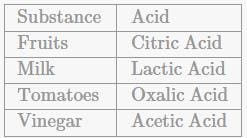RRB JE CE (CBT I) Mock Test- 2 - Question 84

Which of the following makes up to 60%-75% by mass of human body?

Detailed Solution for RRB JE CE (CBT I) Mock Test- 2 - Question 84
Up to 60%- 75% of the human adult body is water.

The brain and heart are composed of 73% water, and the lungs are about 83% water. The skin contains 64% water, muscles and kidneys are 79%, and even the bones are watery: 31%.

Water serves several essential functions to keep us all going:

- A vital nutrient to the life of every cell, acts first as a building material.

- It regulates our internal body temperature by sweating and respiration

- The carbohydrates and proteins that our bodies use as food are metabolized and transported by water in the bloodstream;

- It assists in flushing waste mainly through urination

- acts as a shock absorber for brain, spinal cord, and foetus, forms saliva & lubricates joints

RRB JE CE (CBT I) Mock Test- 2 - Question 85

For a pendulum in Simple Harmonic Motion, Time period (t) is _______________ to Acceleration due to gravity (g).

Detailed Solution for RRB JE CE (CBT I) Mock Test- 2 - Question 85
A simple pendulum is one which can be a point mass suspended from a string or rod of negligible mass. It is a resonant system with a single resonant frequency. For small amplitudes, the period of such a pendulum can be approximated by

T=2π√(l/g)

RRB JE CE (CBT I) Mock Test- 2 - Question 86

_______________ is a process in which two or more metal items are joined together.

Detailed Solution for RRB JE CE (CBT I) Mock Test- 2 - Question 86
Soldering is a process in which two or more metal items are joined together by melting and then flowing a filler metal into the joint - the filler metal having a relatively low melting point. Soldering is used to form a permanent connection between electronic components.
RRB JE CE (CBT I) Mock Test- 2 - Question 87

Current in a 60 W light bulb when it is connected to a 240 V power supply is___________.

Detailed Solution for RRB JE CE (CBT I) Mock Test- 2 - Question 87
According to Ohm's law, Ohm's law equation (formula): V = I X R and the power law equation (formula): P = I x V.

P = power

So, 60 = I x 240

I = 60/240

Therefore, I = 0.25 A

RRB JE CE (CBT I) Mock Test- 2 - Question 88

_____________ is a thermodynamic process in which there is no heat transfer into or out of a system and is generally obtained by surrounding the entire system with a strongly insulating material.

Detailed Solution for RRB JE CE (CBT I) Mock Test- 2 - Question 88
An adiabatic process is a thermodynamic process in which there is no heat transfer into or out of a system and is generally obtained by surrounding the entire system with a strongly insulating material or by carrying out the process so quickly that there is no time for a significant heat transfer to take place.

A system that expands under adiabatic conditions does positive work, so the internal energy decreases, and a system that contracts under adiabatic conditions does negative work, so the internal energy increases.

RRB JE CE (CBT I) Mock Test- 2 - Question 89

Medulla is part of ________ in the human body.

Detailed Solution for RRB JE CE (CBT I) Mock Test- 2 - Question 89
Medulla oblongata, also called medulla, the lowest part of the brain and the lowest portion of the brainstem. The medulla oblongata is connected by the pons to the midbrain and is continuous posteriorly with the spinal cord, with which it merges at the opening (foramen magnum) at the base of the skull.
RRB JE CE (CBT I) Mock Test- 2 - Question 90

_________ is a measuring instrument used to measure the current in a circuit.

Detailed Solution for RRB JE CE (CBT I) Mock Test- 2 - Question 90
The instrument which measures the flows of current in ampere is known as ampere meter or ammeter.

A voltmeter is an electronic instrument used to measure potential between any two points in an electric or electronic circuit in volts

Odometers are used to calculate distance travelled in a vehicle, used along speedometers.

RRB JE CE (CBT I) Mock Test- 2 - Question 91

Which of the following are TRUE regarding the variation of "g "(acceleration due to gravity)?

Detailed Solution for RRB JE CE (CBT I) Mock Test- 2 - Question 91
Acceleration due to gravity is zero at the centre of the earth. The value of g is least at the equator and maximum at the poles. It means the value of acceleration due to gravity increases as we go from the equator to the poles.

The acceleration due to gravity:

- Decreases on account of rotation of the earth.

- Increases with the increase in the latitude of the place.

RRB JE CE (CBT I) Mock Test- 2 - Question 92

Which of the following is related to the statement that "For a given colour of light, the ratio of the sine of the angle of incident and angle of refraction is same"?

Detailed Solution for RRB JE CE (CBT I) Mock Test- 2 - Question 92
Snell's law, in optics, a relationship between the path taken by a ray of light in crossing the boundary or surface of separation between two contacting substances and the refractive index of each. It states that for a given color of light, the ratio of the sine of the angle of incident and angle of refraction is same.

Kirchhoff's current law states that current flowing into a node (or a junction) must be equal to the current flowing out of it. This is a consequence of charge conservation. Kirchhoff's voltage law states that the sum of all voltages around any closed loop in a circuit must equal zero.

Stefan-Boltzmann law, statement that the total radiant heat energy emitted from a surface is proportional to the fourth power of its absolute temperature.

RRB JE CE (CBT I) Mock Test- 2 - Question 93

Plasma is a component of which among the following part of human body?

Detailed Solution for RRB JE CE (CBT I) Mock Test- 2 - Question 93
Plasma is the largest component of your blood, making up about 55% of its overall content. When isolated on its own, blood plasma is a light-yellow liquid, similar to the color of straw. Along with water, plasma carries salts and enzymes.

The primary purpose of plasma is to transport nutrients, hormones, and proteins to the parts of the body that need it. Cells also deposit their waste products into the plasma. The plasma, in turn, helps remove this waste from the body. Blood plasma also ushers the movement of all the elements of blood through the circulatory system.

RRB JE CE (CBT I) Mock Test- 2 - Question 94

Which of the following is defined as the amount of energy released during the formation of one mole of ionic compound from its constituent ions?

Detailed Solution for RRB JE CE (CBT I) Mock Test- 2 - Question 94
Lattice Energy is defined as the amount of energy released during the formation of one mole of ionic compound from its constituent ions.

Lattice energy is relevant to many practical properties including solubility, hardness, and volatility.

Hydration energy is the amount of energy released when one mole of ions undergo hydration which is a special case of solvation. It is a special case of dissolution of energy, with the solvent being water.

RRB JE CE (CBT I) Mock Test- 2 - Question 95

__________ is the speed that an object needs to be traveling to break free of a planet or moon's gravity well and leave it without further propulsion.

Detailed Solution for RRB JE CE (CBT I) Mock Test- 2 - Question 95
Escape velocity is the speed that an object needs to be traveling to break free of a planet or moon's gravity well and leave it without further propulsion. For example, a spacecraft leaving the surface of Earth needs to be going 7 miles per second, or nearly 25,000 miles per hour to leave without falling back to the surface or falling into orbit.

Since escape velocity depends on the mass of the planet or moon that a spacecraft is blasting off of, a spacecraft leaving the moon's surface could go slower than one blasting off of the Earth, because the moon has less gravity than the Earth.

RRB JE CE (CBT I) Mock Test- 2 - Question 96

___________is the study of humans and human behaviour and societies in the past and present.

Detailed Solution for RRB JE CE (CBT I) Mock Test- 2 - Question 96
Anthropology is the study of people throughout the world, their evolutionary history, how they behave, adapt to different environments, communicate and socialise with one another. The study of anthropology is concerned both with the biological features that make us human (such as physiology, genetic makeup, nutritional history and evolution) and with social aspects (such as language, culture, politics, family and religion).

Seismology is the study of earthquakes and seismic waves that move through and around the earth

Psychology is the scientific study of the mind and behaviour. Psychology is a multifaceted discipline and includes many sub-fields of study such areas as human development, sports, health, clinical, social behavior and cognitive processes.

RRB JE CE (CBT I) Mock Test- 2 - Question 97

Which of these is/are applications of Ultrasonic Waves ?

Detailed Solution for RRB JE CE (CBT I) Mock Test- 2 - Question 97
Sound waves with frequencies higher than the upper audible limit of human hearing are called ultrasound. The limit varies from person to person but is approximately 20,000 Hertz. The physical properties of ultrasound are like the normal audible sound

Applications include-

- Measuring depth of sea (SONAR)

- Sterilizing Medical equipment

- Cleaning the various industry specific Machinery parts

RRB JE CE (CBT I) Mock Test- 2 - Question 98

Which of the following have a pH value less than 7?

Detailed Solution for RRB JE CE (CBT I) Mock Test- 2 - Question 98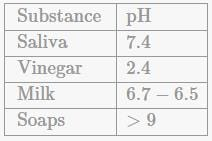RRB JE CE (CBT I) Mock Test- 2 - Question 99

Lakes and forests are best classified as examples of _________

Detailed Solution for RRB JE CE (CBT I) Mock Test- 2 - Question 99
A natural ecosystem is the result of interactions between organisms and the environment. For example, an ocean, lakes, forests etc.

An artificial ecosystem is not self-sustaining, and the ecosystem would perish without human assistance. For example, a farm, an aquarium etc.

The biosphere is a global ecosystem composed of living organisms (biota) and the abiotic (non-living) factors from which they derive energy and nutrients extending from a few kilometres into the atmosphere to the deep-sea vents of the ocean.

RRB JE CE (CBT I) Mock Test- 2 - Question 100

Gammaxene, D.D.T. and Bleaching powder are important compounds of :

Detailed Solution for RRB JE CE (CBT I) Mock Test- 2 - Question 100
These are the compounds of chlorine.
Information about RRB JE CE (CBT I) Mock Test- 2 Page
In this test you can find the Exam questions for RRB JE CE (CBT I) Mock Test- 2 solved & explained in the simplest way possible. Besides giving Questions and answers for RRB JE CE (CBT I) Mock Test- 2, EduRev gives you an ample number of Online tests for practice(Scan QR code)